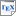[CKLPPW+19] Luca Cardelli, Marta Kwiatkowska, Luca Laurenti, Nicola Paoletti, Andrea Patane, and Matthew Wicker. Statistical Guarantees for the Robustness of Bayesian Neural Networks. In Proc. International Joint Conference on Artificial Intelligence (IJCAI-19). To appear. 2019. [pdf] [bib] Downloads:pdf (8.33 MB)bib Links: [Google] [Google Scholar] [CiteSeer] Abstract. We introduce a probabilistic robustness measure for Bayesian Neural Networks (BNNs), defined as the probability that, given a test point, there exists a point within a bounded set such that the BNN prediction differs between the two. Such a measure can be used, for instance, to quantify the probability of the existence of adversarial examples. Building on statistical verification techniques for probabilistic models, we develop a framework that allows us to estimate probabilistic robustness for a BNN with statistical guarantees, i.e., with a priori error and confidence bounds. We provide experimental comparison for several approximate BNN inference techniques on image classification tasks associated to MNIST and a two-class subset of the GTSRB dataset. Our results enable quantification of uncertainty of BNN predictions in adversarial settings.